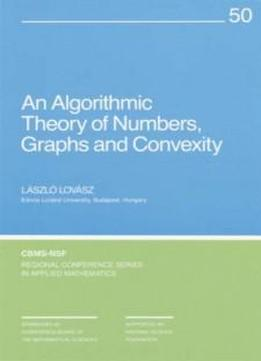# An Algorithmic Theory Of Numbers, Graphs And Convexity (cbms-nsf Regional Conference Series In Applied Mathematics) by Laszlo Lovasz / 1987 / English / PDF

A study of how complexity questions in computing interact with classical mathematics in the numerical analysis of issues in algorithm design. Algorithmic designers concerned with linear and nonlinear combinatorial optimization will find this volume especially useful. Two algorithms are studied in detail: the ellipsoid method and the simultaneous diophantine approximation method. Although both were developed to study, on a theoretical level, the feasibility of computing some specialized problems in polynomial time, they appear to have practical applications. The book first describes use of the simultaneous diophantine method to develop sophisticated rounding procedures. Then a model is described to compute upper and lower bounds on various measures of convex bodies. Use of the two algorithms is brought together by the author in a study of polyhedra with rational vertices. The book closes with some applications of the results to combinatorial optimization.

A study of how complexity questions in computing interact with classical mathematics in the numerical analysis of issues in algorithm design. Algorithmic designers concerned with linear and nonlinear combinatorial optimization will find this volume especially useful. Two algorithms are studied in detail: the ellipsoid method and the simultaneous diophantine approximation method. Although both were developed to study, on a theoretical level, the feasibility of computing some specialized problems in polynomial time, they appear to have practical applications. The book first describes use of the simultaneous diophantine method to develop sophisticated rounding procedures. Then a model is described to compute upper and lower bounds on various measures of convex bodies. Use of the two algorithms is brought together by the author in a study of polyhedra with rational vertices. The book closes with some applications of the results to combinatorial optimization.

views: 487